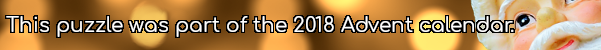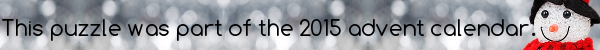mscroggs.co.uk
mscroggs.co.uksubscribe

# Puzzles

## 19 DecemberToday's number is the number of 6-dimensional sides on a 8-dimensional hypercube.
Tags: 3d shapes

## Cube multiples

Six different (strictly) positive integers are written on the faces of a cube. The sum of the numbers on any two adjacent faces is a multiple of 6.
What is the smallest possible sum of the six numbers?

## 3 DecemberWhat is the volume of the smallest cube inside which a rectangular-based pyramid of volume 266 will fit?

## 21 DecemberThis year, I posted instructions for making a dodecahedron and a stellated rhombicuboctahedron.
To get today's number, multiply the number of modules needed to make a dodecahedron by half the number of tube maps used to make a stellated rhombicuboctahedron.

## 2009

2009 unit cubes are glued together to form a cuboid. A pack, containing 2009 stickers, is opened, and there are enough stickers to place 1 sticker on each exposed face of each unit cube.
How many stickers from the pack are left?

## Folding tube maps

Back in 2012, I posted instructions for folding a tetrahedron from tube maps. When tube maps are used, the sides of the tetrahedron are not quite equal. What ratio would the rectangular maps need to be in to give a regular tetrahedron?

## Colliding parallel people

If two people stand 1km apart and walk in the same direction, how far will the have to walk until they collide due to the curvature of the Earth? (diameter of Earth = 12,742km)

## Pyramid and tetrahedron

Source: MathsJam
If four equal equilateral triangles form the sides of a square-based pyramid, what is the ratio of the volume of the pyramid to the volume of the tetrahedron whose sides are the four triangles?

## Archive

Show me a random puzzle
▼ show ▼